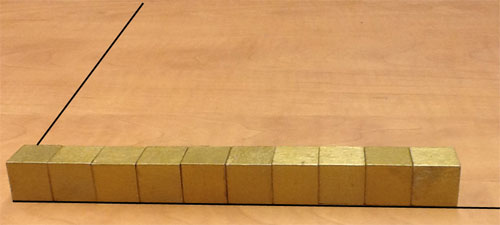SEARCH HOMEMath Central Quandaries & QueriesQuestion from merylin, a student: How many cubic centimeters are in a cubic meter? Use a sketch to explain your reasoning.Hi Merylin,

Draw a 1 meter square on the floor. Cover the 1 meter square region with 1 centimeter cubes, starting along one edge of the 1 meter square and placing a row if 1 centimeter cubes. How many cubes do you need for this row?Place a second row of cubes behind the first row. Continue until you have covered the 1 meter square region. How many rows of cubes do you need? How many cubes did it take?

Now place a second layer of cubes on the first and repeat until you have built a structure that is 1 meter high? How many layers do you need? How many 1 centimeter cubes did you use to build this 1 meter cube structure?

PennyMath Central is supported by the University of Regina and The Pacific Institute for the Mathematical Sciences.